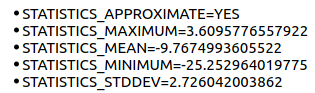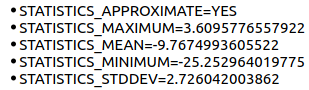# RDTC S1 Scene Not Overlapping on OSM in QGIS

When I import the range doppler terrain corrected S1 scene (saved as geotiff) in QGIS and add a OSM as base layer the features don’t overlap unlike the basemap in SNAP.

Some snapshots for reference.
In QGIS

In SNAP

Looking forward.

you can directly load the img file of the terrain corrected into QGIS. You find it inside the .data folder of the BEAM DIMAP product. Please give it a try.

Thanks Andreas. This works.
But why not geotiffthe Geotiff format has its own structure, but it should be able to store correct geolocation. Don’t kow why it failed in your case, but genereally any conversion between formats can introduce errors and should be avoided if possible.

Although you are right that not all conversions will preserve the image statistics, I don’t see any change in the image statistics when I export the .img file and save it as a geotiff image in QGIS.

For reference:
a) .img image statsb) geotiff image statsI still wonder why the export from SNAP as a geotiff didn’t preserve the correct geolocationsThe adoption by QGIS of new GDAL and PROJ versions has not been smooth: QGIS Public Service Announcement. There are known issues loading GeoTIFF files in some QGIS versions.

1 Like

can you please give me the product ID and all steps you undertook, so I can replicate this error?

I also tried to generate the geotiff with the following script. Surprisingly the output has the same issue as the geotiff exported directly from SNAP.

Here is the script.

###############################Creating Geotiff############################

# Initialize the Image Size

h = 21184 # rows
w = 36334 # columns
image_size = (h,w)

# Geographic info

lat = latitude_array
lon = longitude_array

# Channel to write

r_pixels = Gamma0_VV_array # Gamma0_VV_array in dB

# set geotransform

nx = image_size
ny = image_size
xmin, ymin, xmax, ymax = [np.min(lon[np.nonzero(lon)]), np.min(lat[np.nonzero(lat)]), np.max(lon[np.nonzero(lon)]), np.max(lat[np.nonzero(lat)])]
print(np.min(lon[np.nonzero(lon)]))
print(np.min(lat[np.nonzero(lat)]))
print(np.max(lon[np.nonzero(lon)]))
print(np.max(lat[np.nonzero(lat)]))
xres = (xmax - xmin) / float(nx)
yres = (ymax - ymin) / float(ny)
print(xres)
print(yres)
geotransform = (xmin, xres, 0, ymax, 0, -yres)

# create the 1-band raster file

dst_ds = gdal.GetDriverByName(‘GTiff’).Create(‘myGeoTIFF.tif’, ny, nx, 1, gdal.GDT_Float32)

dst_ds.SetGeoTransform(geotransform) # specify coords
srs = osr.SpatialReference() # establish encoding
srs.ImportFromEPSG(4326) # WGS84 lat/long
dst_ds.SetProjection(srs.ExportToWkt()) # export coords to file
dst_ds.GetRasterBand(1).WriteArray(r_pixels) # write Gamma0_VV_array in dB to the raster

dst_ds.FlushCache() # write to disk
dst_ds = None

################################ Reading the Geotiff and Plotting it on a Basemap##########

import georaster
import matplotlib.pyplot as plt
from mpl_toolkits.basemap import Basemap

fig = plt.figure(figsize=(8,8))

# full path to the geotiff file

fpath = r"myGeoTIFF.tif"

# grab limits of image’s extent

minx, maxx, miny, maxy = my_image.extent

# set resolution at intermediate level “i”

m = Basemap( projection=‘cyl’,
llcrnrlon=minx-2,
llcrnrlat=miny-2,
urcrnrlon=maxx+2,
urcrnrlat=maxy+2,
resolution=‘i’)

m.drawcoastlines(color=“gray”)

# load the geotiff image, assign it a variable

image = georaster.SingleBandRaster( fpath,
latlon=True)

# set alpha to let the hidden graphics show through

plt.imshow(image.r, extent=(minx, maxx, miny, maxy), zorder=10, alpha=0.6)

plt.show()

The following processing steps were followed with the S1 data
S1A_IW_GRDH_1SSV_20160412T140707_20160412T140732_010789_0101FE_8E58

-Thermal noise removal
-Apply orbit file
-Calibration to beta0
-Radiometric terrain flattening (with SRTM DEM)
-Speckle filtering (optional)
-Range doppler terrain correction (RDTC)
-Linear to dB (for Gamma0 VV)

Post this I exported the Gamma0_VV_dB band as GeoTiff/BigTiff. I also tried creating the geotiff with a script (that I replied to @gnwiii) but it had the same geolocation issue.

What QGIS version are you using?

I tested your dataset and get the same results for both img and GeoTiff (didn’t use the BigTiff export).

GeoTiff

img from BEAM DIMAP

1 Like

3.6.2-Noosa